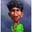Related Tags

math.ceil
math.floor
math.round
javascript
community creator

# Math.ceil, Math.floor, and Math.round in JavaScriptSubha Chanda

## Introduction

We all have learned about rounding off numbers in school. We usually increase the integer if its value is >=.5 and decrease it if its value is <= .4.

1.5 ≈ 2

1.4 ≈ 1

JavaScript has a built-in object called Math that has properties and methods for mathematical constants and functions.

We have three methods that are mostly used to round off a number in JS, i.e., Math.ceil(), Math.floor(), and Math.round(). Let’s explore them in this article.

## Math.ceil

The Math.ceil function in JavaScript is used to round up a number that is passed into it to its nearest integer. What do I mean by rounding up? I mean towards the greater value. Math.ceil() only takes one parameter, the value to be rounded. So, if we have a value of 1.4, Math.ceil() will round off it to 2.

console.log(Math.ceil(1.4));
//2
console.log(Math.ceil(1.6));
//2
console.log(Math.round(-1.4));
//-1
console.log(Math.round(-1.6));
//-1Photo from Wikipedia

## Math.floor()

While the Math.ceil method returns the smallest integer greater than or equal to the value we pass, Math.floor returns the largest or equal integer that is less than the given value. It also takes a single parameter.Photo from Wikipedia

So, if we pass the value 1.4 in Math.floor, we’ll get 1 in return. If we pass 1.6, we’ll also get 1.

console.log(Math.floor(1.4));
// 1
console.log(Math.floor(1.6));
// 1
console.log(Math.floor(-1.4));
// -2
console.log(Math.floor(-1.6));
// -2

## Math.round()

Math.round() rounds off a number depending on the fractional part of the number. So, if the fractional part is >=.5, it’ll return the smallest integer that is still greater than the passed value. If the number is <=.4, we’ll get the largest possible integer that is still smaller than the number we passed.

console.log(Math.round(1.4));
// 1
console.log(Math.round(1.6));
// 2
console.log(Math.round(1.5));
// 2
console.log(Math.round(-1.4));
// -1
console.log(Math.round(-1.6));
// -2
console.log(Math.round(-1.5));
// -1

So, Math.round() can go both upward and downward depending on the fractional part.## Math.trunc()

There’s another method available in JS Math object, Math.trunc(). Math.trunc() returns the integer part of a number by removing any fractional part of that number.

console.log(Math.trunc(1.4));
// 1
console.log(Math.trunc(1.6));
// 1
console.log(Math.trunc(-1.4));
// -1
console.log(Math.trunc(-1.6));
// -1

## Conclusion

Rounding off numbers is an essential part of programming. I hope this article triggered your memory of the different built-in rounding off methods we have in JavaScript. Leave an ❤ if you found this article helpful. 😊

RELATED TAGS

math.ceil
math.floor
math.round
javascript
community creator

CONTRIBUTORSubha Chanda
RELATED COURSES

View all Courses

Keep Exploring

Learn in-demand tech skills in half the time